Examples

Chapter 9 Class 9 - Areas of Parallelograms and Triangles [Deleted]
Serial order wise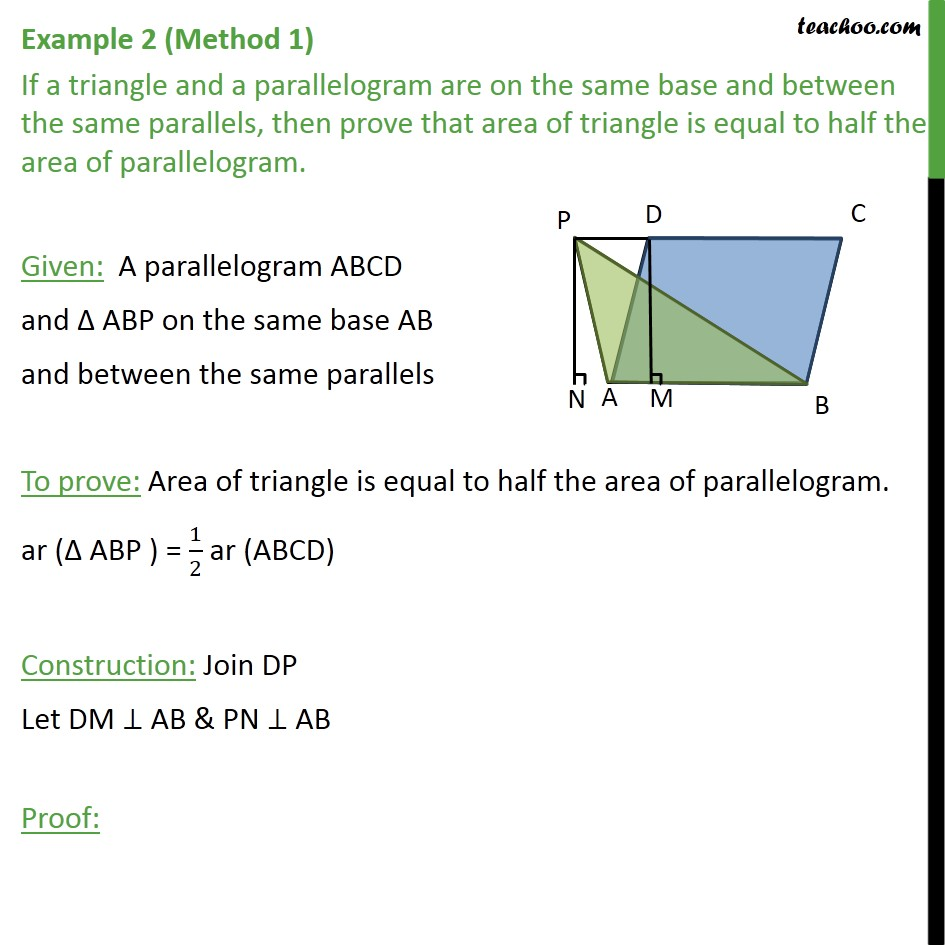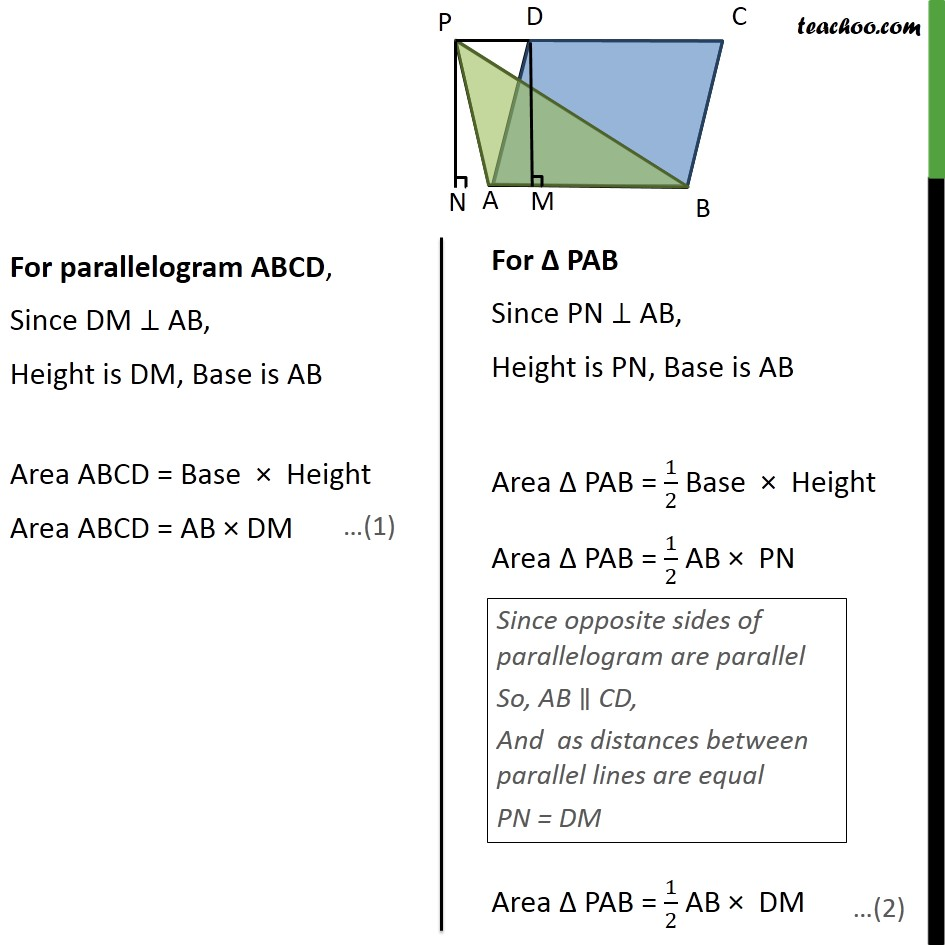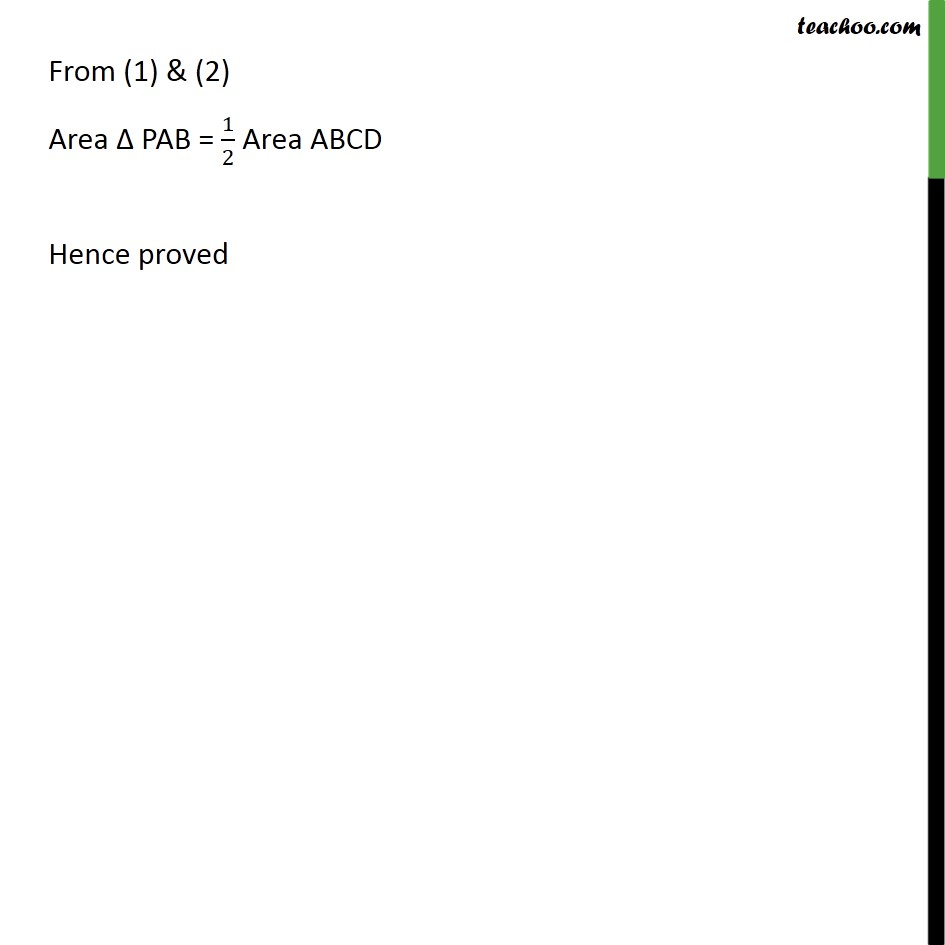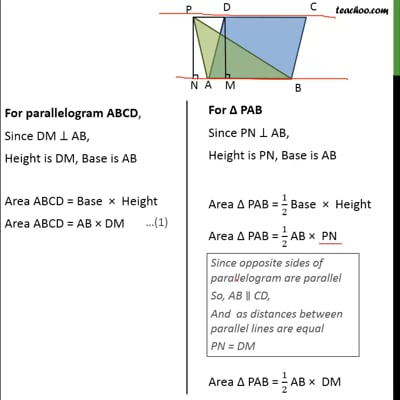This video is only available for Teachoo black users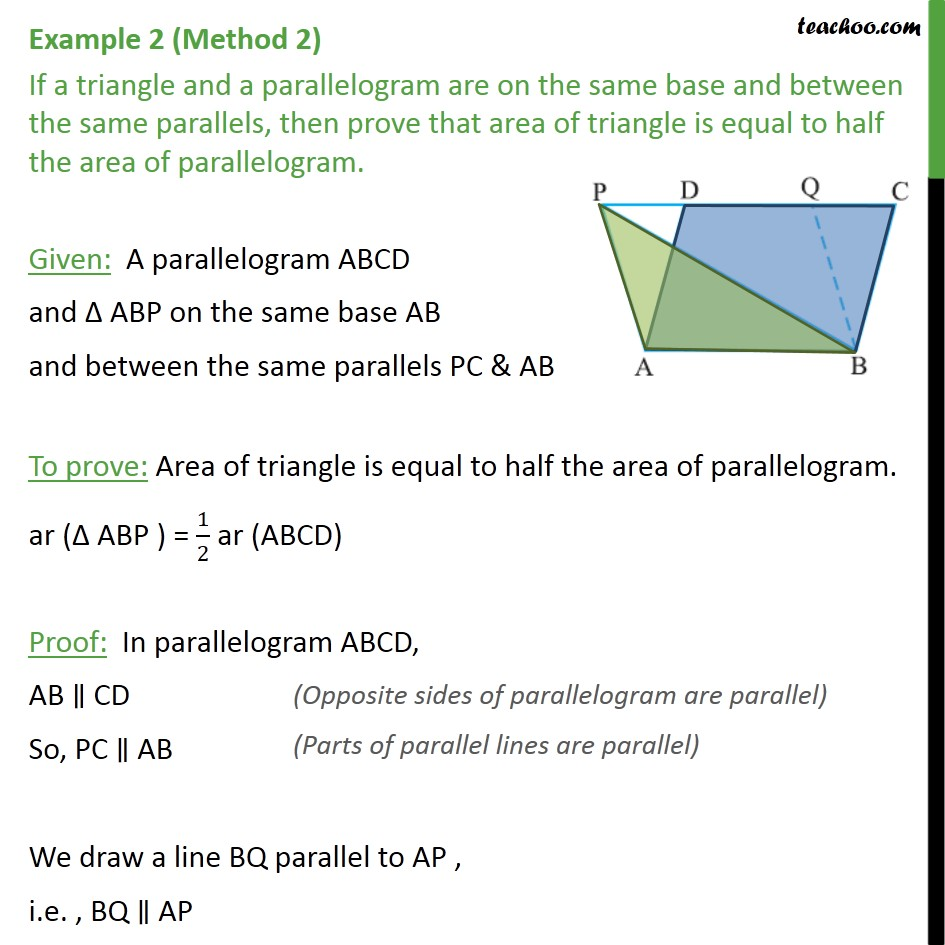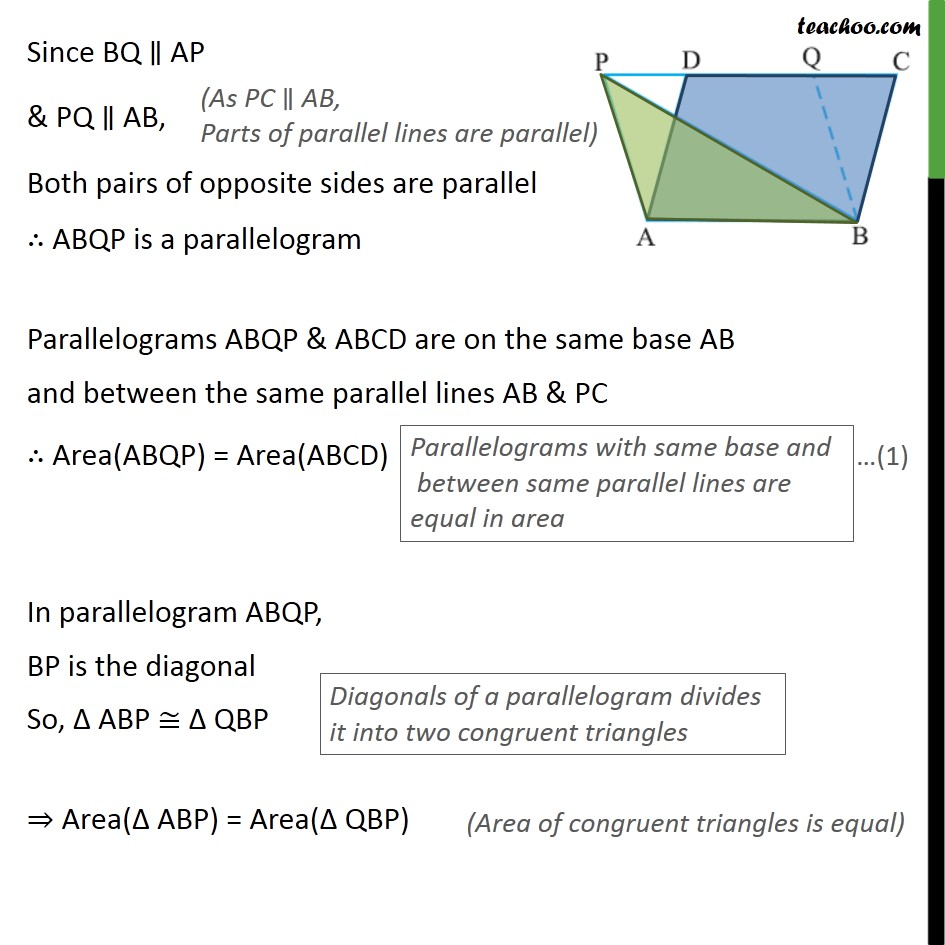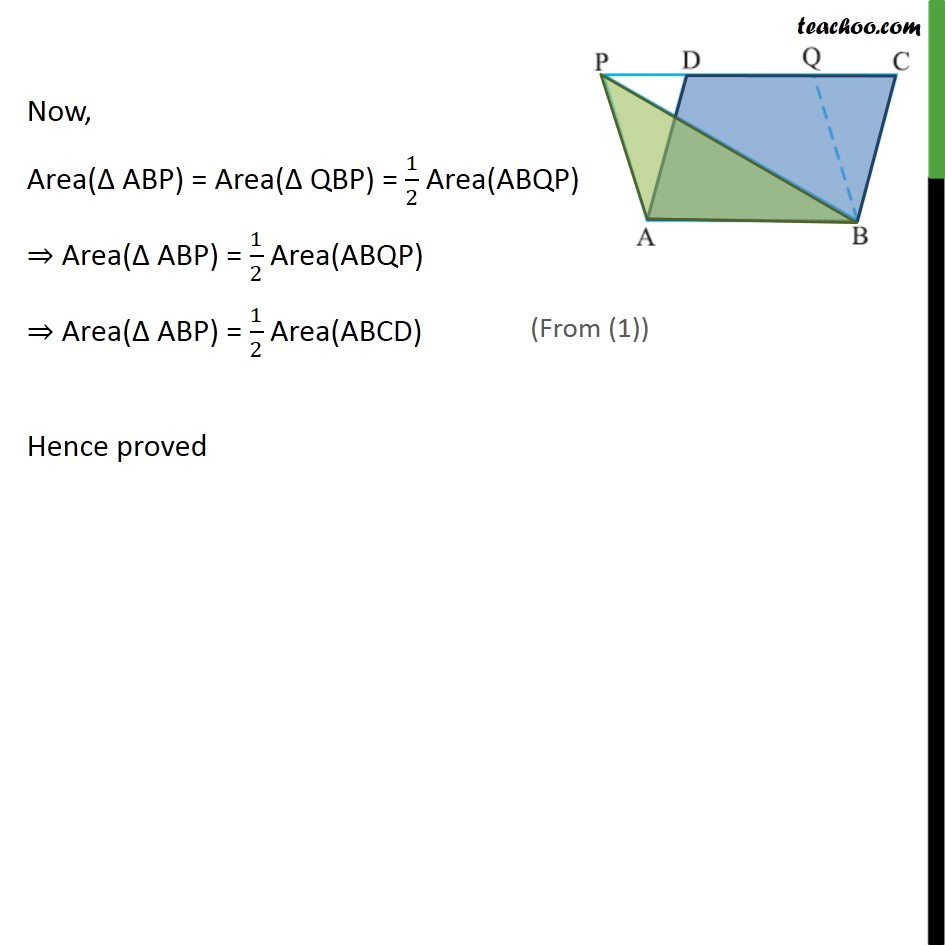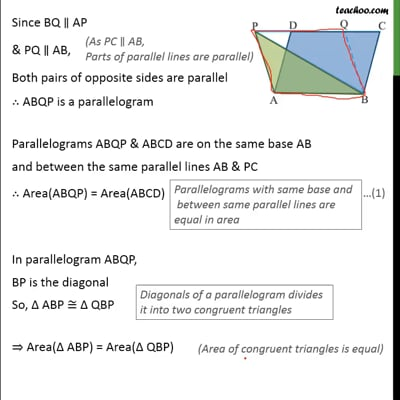This video is only available for Teachoo black users

Solve all your doubts with Teachoo Black (new monthly pack available now!)

### Transcript

Example 2 (Method 1) If a triangle and a parallelogram are on the same base and between the same parallels, then prove that area of triangle is equal to half the area of parallelogram. Given: A parallelogram ABCD and ABP on the same base AB and between the same parallels To prove: Area of triangle is equal to half the area of parallelogram. ar ( ABP ) = 1/2 ar (ABCD) Construction: Join DP Let DM AB & PN AB Proof: Example 2 (Method 2) If a triangle and a parallelogram are on the same base and between the same parallels, then prove that area of triangle is equal to half the area of parallelogram. Given: A parallelogram ABCD and ABP on the same base AB and between the same parallels PC & AB To prove: Area of triangle is equal to half the area of parallelogram. ar ( ABP ) = 1/2 ar (ABCD) Proof: In parallelogram ABCD, AB CD So, PC AB We draw a line BQ parallel to AP , i.e. , BQ AP Since BQ AP & PQ AB, Both pairs of opposite sides are parallel ABQP is a parallelogram Parallelograms ABQP & ABCD are on the same base AB and between the same parallel lines AB & PC Area(ABQP) = Area(ABCD) In parallelogram ABQP, BP is the diagonal So, ABP QBP Area( ABP) = Area( QBP) Now, Area( ABP) = Area( QBP) = 1/2 Area(ABQP) Area( ABP) = 1/2 Area(ABQP) Area( ABP) = 1/2 Area(ABCD) Hence proved